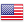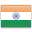Grade "A+" Accredited by NAAC with a CGPA of 3.46
Grade "A+" Accredited by NAAC with a CGPA of 3.46

Course ID
MC 103
Level
Program
BMS
Semester
First
Credits
6.0
Paper Type
Core Course
Method
Lecture & Tutorial

## Unique Paper Code: 61011104

To familiarize the students with various Statistical Data Analysis tools that can be used for effective decision making. Emphasis will be on the application of the concepts learnt to various managerial situations.

## Learning Outcomes:

At the end of the course, students should be able to:

• Summarize data sets using Descriptive statistics
• Analyze the relationship between two variables
• Analyze trend and seasonality in a time series data
• Draw conclusion about a population using testing of hypothesis
• Apply statistics to different managerial situations

## Course Contents

Unit I
Unit II
Unit III
Unit IV

Unit I (3 Weeks)
Data: Types and sources, quantitative and qualitative, attributes, Big Data and its utility in business analytics, Scales of measurement: nominal, ordinal, interval and ratio, Measures of Central Value: Mean , Median and Mode, Measures of Dispersion: Absolute and Relative measures of dispersion – Range, Quartile Deviation, Mean Deviation, Standard Deviation, Coefficient of Variation, Box and whisker plot, Moments, Shape of the distribution: Skewness and Kurtosis, z-score, Chebychev and empirical rule.

References:
Stine and Foster, Statistics for Business (Decision making and Analysis), Pearson [Chapter 2, 3, 4]
S.P. Gupta, Statistical Methods, Sultan Chand & Sons [Vol I, Chapter 5,6,7,8 and 9]

Unit II (2 Weeks)
Correlation Analysis: Meaning and significance. Correlation and Causation, Types of correlation, Methods of studying simple correlation – Scatter diagram, Karl Pearson’s coefficient of correlation, Spearman’s Rank correlation coefficient, Regression Analysis: Meaning and significance, Regression vs. Correlation, Simple Regression model:Linear Regression, Conditions for simple linear regression, Standard error of estimate, Tests for the significance of correlation coefficient

References:
S.P. Gupta, Statistical Methods, Sultan Chand & Sons [Vol I, Chapter 10, 11]
Stine and Foster, Statistics for Business (Decision making and Analysis), Pearson [Chapter 21]

Unit III (3 Weeks)
Analysis of Time Series: Meaning and significance, Utility, Components of time series, Models (Additive and Multiplicative), Measurement of trend: Method of least squares, Linear and Parabolic trend, Measurement of seasonal variations by method of ratio to trend.

Index Numbers: Meaning and significance, problems in construction of index numbers, Methods of constructing index numbers-weighted and unweighted, Construction of index numbers of prices and quantities, Consumer price index number, Introduction to stock market index

References:
S.P. Gupta, Statistical Methods, Sultan Chand & Sons [Vol I, Chapter 13, 14] Levin and Rubin, Statistics for Management, Pearson. [Chapter 15, 16]

Unit IV (4 Weeks)
Probability: Meaning and need, Theorems of addition and multiplication, Conditional probability, Bayes’ theorem, Random Variable- discrete and continuous. Probability Distribution: Meaning, characteristics (Expectation and variance) of Binomial, Poisson, exponential and Normal distribution, Central limit theorem.

Estimation of population mean, Confidence intervals for the parameters of a normal distribution (one sample only), Introduction to testing of Hypothesis: Concept; Level of Significance; Process of testing; Type I and Type II error, Test of hypothesis concerning Mean: z test & t test.

References:
Keller, G, Statistics for Management and Economics, Cengage Learning. [Chapter 6, 7, 8 ] Levin and Rubin, Statistics for Management, Pearson. [Chapter 4, 5, 7, 8]

#### Text Books

Keller, G. (2015). Statistics for Management and Economics. 10thEd. New Delhi: Cengage Learning.
Levin, R. and Rubin, D. (2012). Statistics for Management. 7thEd. New Delhi: Pearson.
Stine, R. and Foster. (2014). Statistics for Business (Decision making and Analysis). 2ndEd. New Delhi: Pearson
Gupta S.P. (2017) : Statistical Methods, Sultan Chand & Sons, 45h Revised Edition

Vohra, N.D. (latest editions) Business Statistics, New Delhi, McGraw Hills

#### Teaching Learning Process

The teaching learning process will consist of imparting key concepts of statistics to the students. To make the lectures interesting use of PPTs and audio visual presentation is advisable as and when needed. The students should be encouraged to solve real life business/ managerial problems through software like MS Excel

#### Assessment Methods

Assessment of the students will take place on a continuous evaluation basis. It will primarily consist of class test, assignment, presentation and attendance.
Internal Assessment: 25 marks (including 5 marks for attendance)
End term written examination: 75 marks

#### Keywords

Descriptive statistics, Inferential statistics, Central Tendency, Measures of Dispersion, Correlation, Regression, Time series, Random variable, Probability distribution, Testing of hypothesis

Disclaimer: Details on this page are subject to change as per University of Delhi guidelines. For latest update in this regard please refer to the University of Delhi website here.Englishहिन्दी